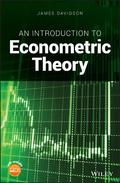John Wiley & Sons An Introduction to Econometric TheoryA guide to economics, statistics and finance that explores the mathematical foundations underling ec.. Product #: 978-1-119-48488-2 Regular price: \$76.10 \$76.10 Auf Lager

# An Introduction to Econometric Theory1. Auflage Oktober 2018
250 Seiten, Hardcover
Wiley & Sons Ltd

ISBN: 978-1-119-48488-2
John Wiley & Sons

Jetzt kaufen

### Preis: 79,90 €

Preis inkl. MwSt, zzgl. Versand

A guide to economics, statistics and finance that explores the mathematical foundations underling econometric methods

An Introduction to Econometric Theory offers a text to help in the mastery of the mathematics that underlie econometric methods and includes a detailed study of matrix algebra and distribution theory. Designed to be an accessible resource, the text explains in clear language why things are being done, and how previous material informs a current argument. The style is deliberately informal with numbered theorems and lemmas avoided. However, very few technical results are quoted without some form of explanation, demonstration or proof.

The author -- a noted expert in the field -- covers a wealth of topics including: simple regression, basic matrix algebra, the general linear model, distribution theory, the normal distribution, properties of least squares, unbiasedness and efficiency, eigenvalues, statistical inference in regression, t and F tests, the partitioned regression, specification analysis, random regressor theory, introduction to asymptotics and maximum likelihood. Each of the chapters is supplied with a collection of exercises, some of which are straightforward and others more challenging. This important text:
* Presents a guide for teaching econometric methods to undergraduate and graduate students of economics, statistics or finance
* Offers proven classroom-tested material
* Contains sets of exercises that accompany each chapter
* Includes a companion website that hosts additional materials, solution manual and lecture slides

Written for undergraduates and graduate students of economics, statistics or finance, An Introduction to Econometric Theory is an essential beginner's guide to the underpinnings of econometrics.

List of Figures ix

Preface xi

Part I Fitting 1

1 Elementary Data Analysis 3

1.1 Variables and Observations 3

1.2 Summary Statistics 4

1.3 Correlation 6

1.4 Regression 10

1.5 Computing the Regression Line 12

1.6 Multiple Regression 16

1.7 Exercises 18

2 Matrix Representation 21

2.1 Systems of Equations 21

2.2 Matrix Algebra Basics 23

2.3 Rules of Matrix Algebra 26

2.4 Partitioned Matrices 27

2.5 Exercises 28

3 Solving the Matrix Equation 31

3.1 Matrix Inversion 31

3.3 Transposes and Products 37

3.4 Cramer's Rule 38

3.5 Partitioning and Inversion 39

3.6 A Note on Computation 41

3.7 Exercises 43

4 The Least Squares Solution 47

4.1 Linear Dependence and Rank 47

4.2 The General Linear Regression 50

4.3 Definite Matrices 52

4.4 Matrix Calculus 56

4.5 Goodness of Fit 57

4.6 Exercises 59

Part II Modelling 63

5 Probability Distributions 65

5.1 A Random Experiment 65

5.2 Properties of the Normal Distribution 68

5.3 Expected Values 72

5.4 Discrete Random Variables 75

5.5 Exercises 80

6 More on Distributions 83

6.1 Random Vectors 83

6.2 The Multivariate Normal Distribution 84

6.3 Other Continuous Distributions 87

6.4 Moments 90

6.5 Conditional Distributions 92

6.6 Exercises 94

7 The Classical RegressionModel 97

7.1 The Classical Assumptions 97

7.2 The Model 99

7.3 Properties of Least Squares 101

7.4 The Projection Matrices 103

7.5 The Trace 104

7.6 Exercises 106

8 The Gauss-Markov Theorem 109

8.1 A Simple Example 109

8.2 Efficiency in the General Model 111

8.3 Failure of the Assumptions 113

8.4 Generalized Least Squares 114

8.5 Weighted Least Squares 116

8.6 Exercises 118

Part III Testing 121

9 Eigenvalues and Eigenvectors 123

9.1 The Characteristic Equation 123

9.2 Complex Roots 124

9.3 Eigenvectors 126

9.4 Diagonalization 128

9.5 Other Properties 130

9.6 An Interesting Result 131

9.7 Exercises 133

10 The Gaussian RegressionModel 135

10.1 Testing Hypotheses 135

10.3 Confidence Regions 140

10.4 t Statistics 141

10.5 Tests of Linear Restrictions 144

10.6 Constrained Least Squares 146

10.7 Exercises 149

11 Partitioning and Specification 153

11.1 The Partitioned Regression 153

11.2 Frisch-Waugh-Lovell Theorem 155

11.3 Misspecification Analysis 156

11.4 Specification Testing 159

11.5 Stability Analysis 160

11.6 Prediction Tests 162

11.7 Exercises 163

Part IV Extensions 167

12 Random Regressors 169

12.1 Conditional Probability 169

12.2 Conditional Expectations 170

12.3 StatisticalModels Contrasted 174

12.4 The Statistical Assumptions 176

12.5 Properties of OLS 178

12.6 The Gaussian Model 182

12.7 Exercises 183

13 Introduction to Asymptotics 187

13.1 The Lawof Large Numbers 187

13.2 Consistent Estimation 192

13.3 The Central LimitTheorem 195

13.4 Asymptotic Normality 198

13.5 Multiple Regression 201

13.6 Exercises 203

14 Asymptotic Estimation Theory 207

14.1 Large Sample Efficiency 207

14.2 Instrumental Variables 208

14.3 Maximum Likelihood 210

14.4 Gaussian ML 213

14.5 Properties of ML Estimators 214

14.6 Likelihood Inference 216

14.7 Exercises 218

Part V Appendices 221

A The Binomial Coefficients 223

B The Exponential Function 225

C Essential Calculus 227

D The Generalized Inverse 229